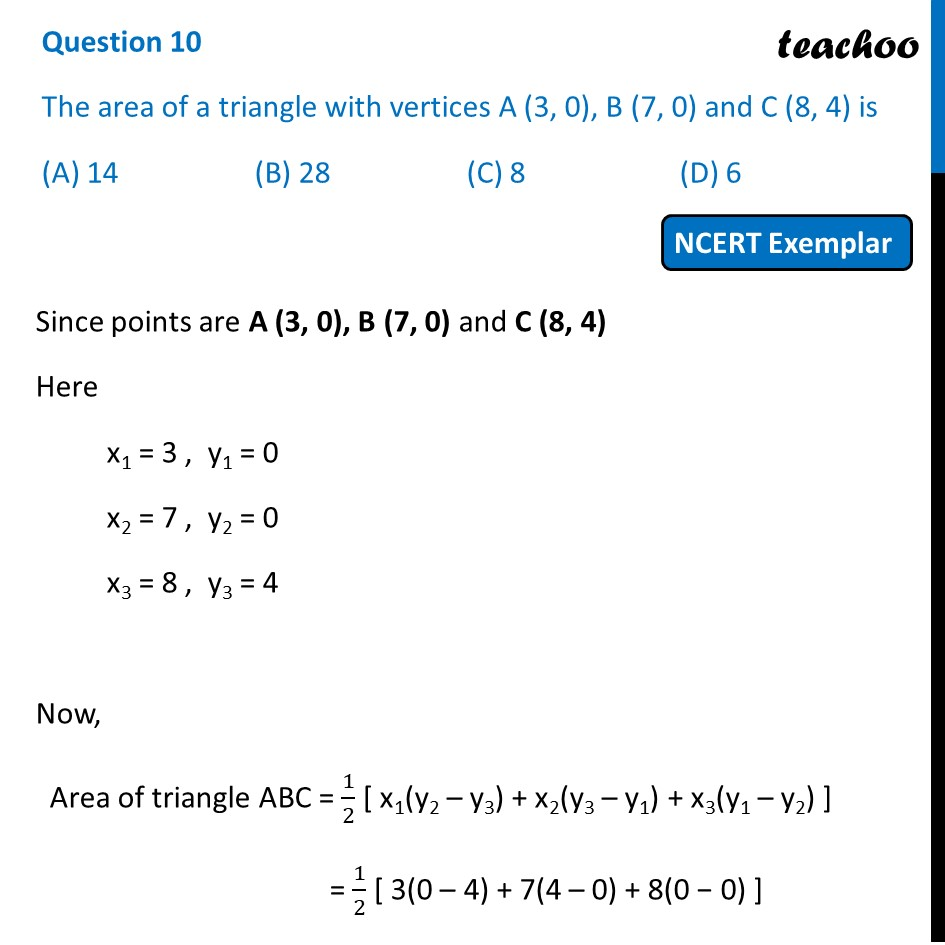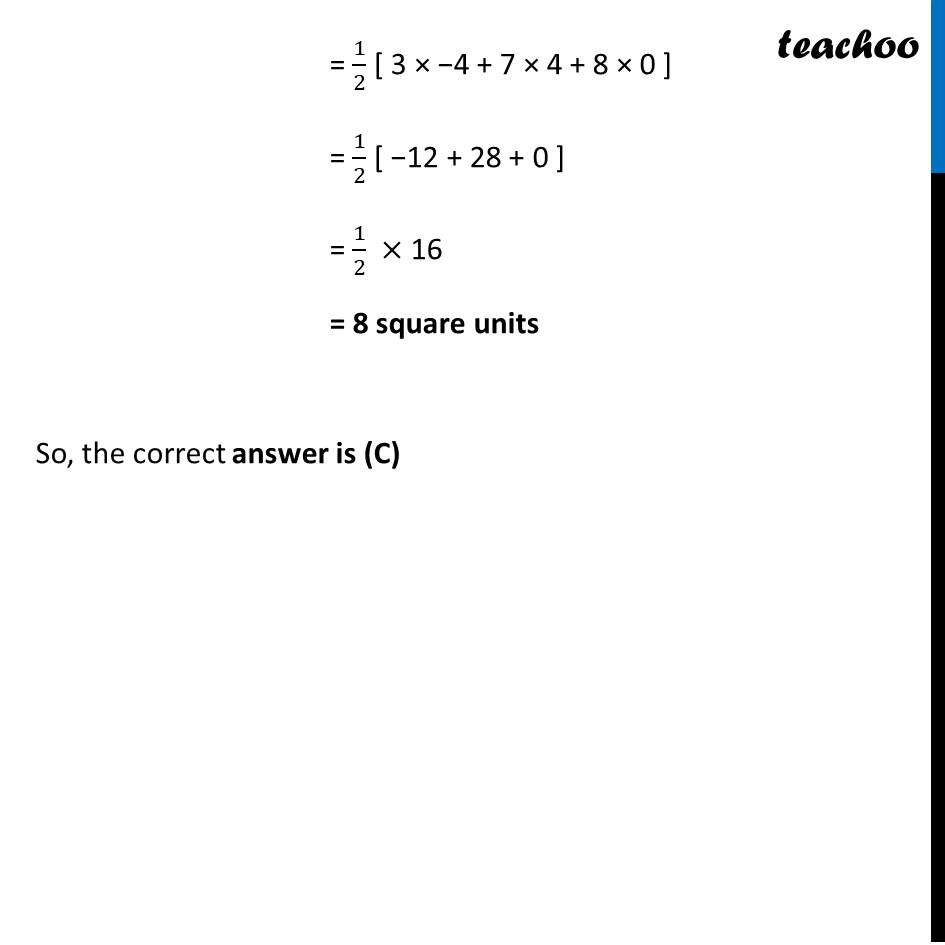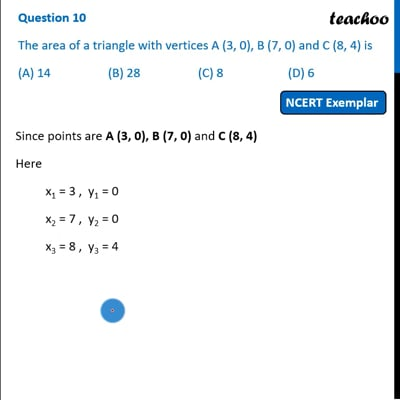NCERT Exemplar - MCQ

Chapter 7 Class 10 Coordinate Geometry
Serial order wise

## (A) 14   (B) 28   (C) 8   (D) 6This video is only available for Teachoo black users

Introducing your new favourite teacher - Teachoo Black, at only ₹83 per month

### Transcript

Question 10 The area of a triangle with vertices A (3, 0), B (7, 0) and C (8, 4) is (A) 14 (B) 28 (C) 8 (D) 6 Since points are A (3, 0), B (7, 0) and C (8, 4) Here x1 = 3 , y1 = 0 x2 = 7 , y2 = 0 x3 = 8 , y3 = 4 Now, Area of triangle ABC = 1/2 [ x1(y2 – y3) + x2(y3 – y1) + x3(y1 – y2) ] = 1/2 [ 3(0 – 4) + 7(4 – 0) + 8(0 − 0) ] = 1/2 [ 3 × −4 + 7 × 4 + 8 × 0 ] = 1/2 [ −12 + 28 + 0 ] = 1/2 × 16 = 8 square units So, the correct answer is (C)